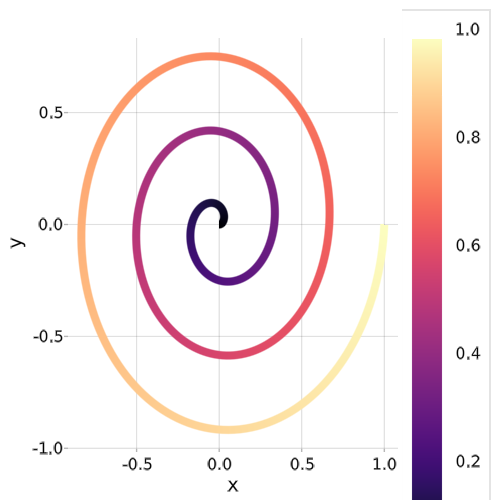## Line with varying colors

```
using ColorSchemes      # colormaps galore

using AbstractPlotting

t = range(0, stop=1, length=500) # time steps

θ = (6π) .* t    # angles

x = t .* cos.(θ) # x coords of spiral
y = t .* sin.(θ) # y coords of spiral

p1 = lines(
x,
y,
color = t,
colormap = ColorSchemes.magma.colors,
linewidth=8)

cm = colorlegend(
p1[end],             # access the plot of Scene p1
raw = true,          # without axes or grid
camera = campixel!,  # gives a concrete bounding box in pixels
# so that the `vbox` gives you the right size
width = (            # make the colorlegend longer so it looks nicer
30,              # the width
540              # the height
)
)

scene_final = vbox(p1, cm) # put the colorlegend and the plot together in a `vbox`

```